In this lesson:

– use perspective mode
– describe frustum
– we use ortho-mode

We turn to 3D. And first let’s figure out how we can realize the prospect. That is, as the distance from us, the objects become smaller, and as they approach – more.

We look at the code, the class OpenGLRenderer. In the method prepareData two vertices are given.

```float x1 = -0.5f, y1 = -0.8f, x2 = 0.5f, y2 = -0.8f;

float[] vertices = {
x1, y1, 0.0f, 1.0f,
x2, y2, 0.0f, 1.0f,
};
```

Note that we use 4 values ​​for each vertex – x, y, z, and w. If everything is clear from x, y, z, it’s just the coordinates of the three axes, then the 4th value (w) is unknown to us, we haven’t used it before. If it is not explicitly included in the vertex data, the default value in the shader will be 1. We will also make it equal to one.

The onDrawFrame method states that we need to draw two points from these vertices

```glDrawArrays(GL_POINTS, 0, 2);
```

let’s launch the application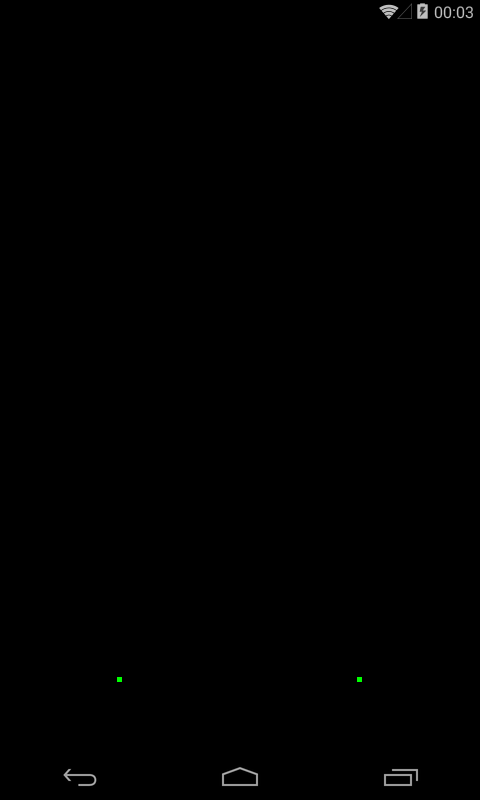There are two green dots on the screen

Now let’s find out why we need this 4th vertex value – w. It is used by the system to create perspective. When the vertex (x, y, z, w) is input to the system, the system divides the coordinates x, y, z by w and eventually receives the vertex by the coordinates (x / w, y / w, z / w), and this division gives perspective effect. Let’s make sure. Rewrite the array to prepareData:

```float[] vertices = {
x1, y1, 0.0f, 1.0f,
x1, y1, 0.0f, 1.5f,
x1, y1, 0.0f, 2.0f,
x1, y1, 0.0f, 2.5f,
x1, y1, 0.0f, 3.0f,
x1, y1, 0.0f, 3.5f,

x2, y2, 0.0f, 1.0f,
x2, y2, 0.0f, 1.5f,
x2, y2, 0.0f, 2.0f,
x2, y2, 0.0f, 2.5f,
x2, y2, 0.0f, 3.0f,
x2, y2, 0.0f, 3.5f,
};
```

We continue to use only two points (x1, y1, 0) and (x2, y2, 0), but now we output each of them 6 times, changing the value of w from 1 to 3.5.

It is logical to assume that if you draw the same point 6 times, the screen will result in one point. But we use different w for each of the 6 points. That is, when drawing each point, its coordinates will be divided by w, and therefore will be different from the others. For example, for a point (x1, y1,0) we get a set of points: (x1 / 1, y1 / 1, 0/1)
(X1 / 1.5, y1 / 1.5, 0 / 1.5)
(X1 / 2, y1 / 2, 0/2)
etc.

That is, they will be completely different points and, accordingly, they will be drawn in different places, not in one.

In the method onDraw don’t forget to specify the glDrawArrays method that we now need to draw not 2 but 12 vertices.

```glDrawArrays(GL_POINTS, 0, 12);
```

run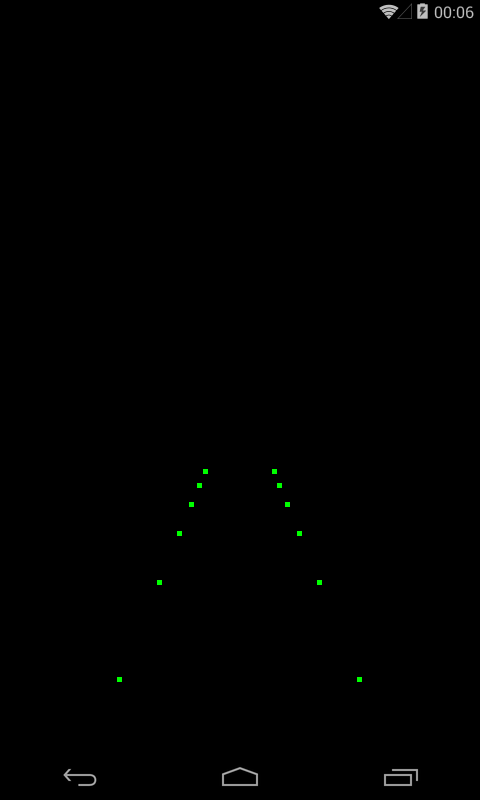Each of the two points has now turned into 6 points. And notice how they are relative to each other. An illusion of perspective is created, that is, the points seem to be moving away from us. As long as you pay attention to the size of the point, it does not change. Look exactly at the placement. The greater the value of w, the “farther” from us is the point in the final image.

When I was studying this topic myself, I had some kind of “pattern break”. I once expected that I would simply set the z-coordinate and, thus, indicate to the system how far or near my point would be. And here is some w.

Let’s try to forget about w and use z.

Rewrite the array to prepareData:

```float[] vertices = {
x1, y1, -1.0f,
x1, y1, -1.5f,
x1, y1, -2.0f,
x1, y1, -2.5f,
x1, y1, -3.0f,
x1, y1, -3.5f,

x2, y2, -1.0f,
x2, y2, -1.5f,
x2, y2, -2.0f,
x2, y2, -2.5f,
x2, y2, -3.0f,
x2, y2, -3.5f,
};
```

And replace the POSITION_COUNT constant from 4 to 3.

```private final static int POSITION_COUNT = 3;
```

This constant is used in the glVertexAttribPointer method and denotes the number of components we use to pass vertex location data. In the previous example we used 4 components (XYZW) and now we will only have 3 (XYZ).

We have removed the w data from the top (it will automatically be 1 when transferring data to the shader). Now we use different z-coordinates. That is, it seems intuitive that approximately the same result should be obtained, that is, the points should be lined up in perspective, further and further, because they are moving away from us at the expense of the z-coordinate.

run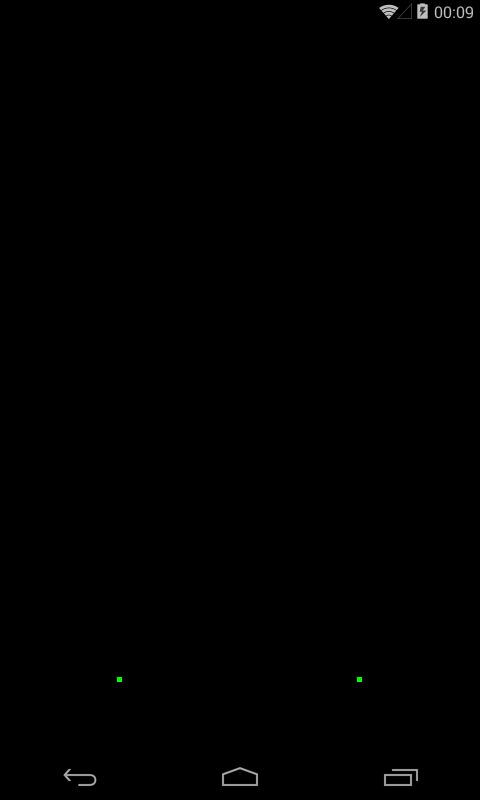We only see two points. The focus failed. And here is one very important thing to understand. Our screen is a two-dimensional image. That is, he has only two axes – X and Y. Accordingly, he takes into account only these coordinates when placing on the screen all objects. And if we want to create the illusion of deleting an object, that is, reducing it in size and some offset along the perspective, then we need to change the X and Y values.

Here is an analogy with a piece of paper. You took a letter and painted a house, for example. And then you were asked to draw exactly the same house, but that it stood a little further “inland” of the letter. You just take and draw the same house, but a little smaller, because it’s located a little “farther” from you, and your brain knows that the distance of an object can be emulated simply by making it smaller. But you did not use any z-coordinates. You painted everything on a 2D sheet and used only the X and Y axes.

The situation is similar with OpenGL. The system expects you to have x and y coordinates to draw them on a two-dimensional screen. And she can depict any perspective of the object, only using the x and y coordinates. We have seen from the example points how the value of w can help us. It changes x and y and gives us perspective on the final image.

But then a reasonable question arises – why do you need the z coordinate at all? There is also a job for her. In our two-dimensional image, it is used by a depth buffer (also called a z-buffer). An example is when you already have two adjacent (x, y) dots at the stage of drawing by the image system. For example: (1,2,0) and (1,2, -1). That is, they both have the coordinates x = 1 and y = 2, but differ only in z. And suppose one of these points is red and the other blue. Which system should draw on the screen?

By default, the last one will be shown. That is, it will simply be drawn on top of the previous one. But this is not always correct in terms of the 3D scene. After all, we can first send a close object to the drawing and then a distant object. And in a proper 3D scene, if both of these objects are in the same line of view, the near object must overlap with the far object. But by default it will be visible far, because it was drawn after the neighbor and just wiped it. And here comes the z-coordinate. It is the depth buffer that determines which of the points is “closer” to you, and which is further, and will display the neighbor. And far away, it will not be drawn accordingly.

It should also be noted here that the z-coordinate is limited to 1 in each direction. That is, all points that have a z-coordinate greater than 1 or less than -1 will simply not be drawn. That is, similar to the coordinates of x and y. If you remember, we talked about it in lesson 169.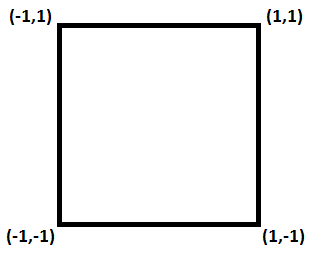That is, all summary points must lie between -1 and +1 on each of the three axes.

When I read up to this point, I was a little upset because it all looks kind of awkward, weird and unclear. Especially the w-value, which has to be calculated in some way in order to set this or that distance of removal of the object.

But! Everything turned out to be not as sad as it might have seemed. OpenGL kindly provides mechanisms that let us know nothing about the w-value and use the same z-coordinate to specify the distance to the object. To do this, we just need to create a matrix and use it.

The main point is that there are two coordinate systems:
1) The first one is the 2D we just looked at. Where a pair of x and y sets the location of a point on the screen, z is used by the depth buffer and w is used to adjust xyz to get perspective. It is on this system that we have worked with you until now.
2) The second coordinate system is virtual 3D space. It has three axes of coordinates, and it uses the xyz coordinates to specify the location of the object. It is on this system that we will create our image. And the system, using a matrix, will convert it all to the first system, that is, to the usual coordinates of the 2D screen.

That is, we will place the vertex in 3D using three coordinates (x, y, z) and pass it to the shader. Also in the shader we will pass the matrix. And the shader with the matrix will transform the vertex and get the output (x, y, z, w) values ​​in which the perspective will already be calculated and these values ​​will be used by the system for drawing. That is, the matrix calculates for us, as from z specified by us, to obtain w so that the object is drawn as if it were at the specified distance (z). Thus, the matrix will transition from a virtual three-dimensional world to a two-dimensional screen.

So, we need to create this magic matrix, pass it to the shader, and then to the shader to use this matrix. Before we create a matrix, we need to understand what it will do. We look at the picture.Camera position is the point where the camera is located. That is from this point we will see the image.

Near plane and Far plane – near and far visibility limits. The camera’s “gaze” direction runs through the center of these borders. Also coming from the camera are four rays that pass through the vertices of these boundaries and eventually form a pyramid. The camera will see everything in this pyramid in between near and far borders (this area is called frustum).

That is, how will the screen image be obtained?

1) First we draw our objects that we want to see. To do this, we always set an array of vertices and ask to draw the objects we need. That is, everything we did before, in the past lessons. The only difference is we will now use the z-coordinate. This will allow us to build a full 3D image, that is, to “zoom in” and “zoom out” objects.

2) We form a frustum matrix, that is, a matrix that will contain data about the pyramid (which we just discussed). To do this, we will specify the distance from the camera to the near and far borders, and the dimensions of the near-border. This will be enough to fully describe the frustum zone.

3) In the shader we apply the matrix of claim 2 to our vertices of claim 1. This will project three-dimensional objects onto a two-dimensional surface. That is, the part of the work I talked about at the beginning of the lesson will be done when the volume moves to the plane and a w-value is used to create perspective and z is used as a z-buffer.

That is, converting virtual 3D coordinates to real 2D coordinates to display images on a two-dimensional screen and maintain 3D visibility.

And as you may recall, we were saying that on the two-dimensional screen we have limits on each of the axes. That is points beyond the coordinates -1 and 1 on each of the three axes that will not be drawn. When converting from a 3D scene to a 2D screen, the Frustum matrix calculates that objects outside the frustum zone will be located at -1 and 1 coordinates after being converted to 2D, and will not be drawn accordingly. .

Let’s go from theory to practice and create a frustum matrix

```attribute vec4 a_Position;
uniform mat4 u_Matrix;

void main()
{
gl_Position = u_Matrix * a_Position;
gl_PointSize = 5.0;
}
```

Previously, we just passed the coordinates of the vertex (a_Position) to the system (gl_Position). Now we will transform them with a matrix that will transform the 3D scene into a 2D screen. To do this, we add the u_Matrix matrix to the shader as a uniform parameter. And we will multiply this matrix by a_Position.

In the method bindData we add code to access the matrix in the shader

```private void bindData(){
// координаты
…

// цвет
…

// матрица
uMatrixLocation = glGetUniformLocation(programId, "u_Matrix");
}
```

Nothing new for us. We use the glGetUniformLocation method and specify the program and the variable name in the shader. And the variable uMatrixLocation has already been declared by me in source codes.

It remains to create a matrix and transfer it to the shader.

Let’s create a method in the same class bindMatrix:

```private void bindMatrix(int width, int height) {
float left = -1.0f;
float right = 1.0f;
float bottom = -1.0f;
float top = 1.0f;
float near = 1.0f;
float far = 8.0f;

Matrix.frustumM(mProjectionMatrix, 0, left, right, bottom, top, near, far);
glUniformMatrix4fv(uMatrixLocation, 1, false, mProjectionMatrix, 0);
}
```

Here we specify all the parameters of the frustum area. near and far is the distance from the camera to the near and far borders. variables left, right, bottom and top are the coordinates of the sides of the near-border. If left = -1 and right = 1, it is easy to calculate that the width of near in our three-dimensional scene will be 2. The same height will be equal to 2 because bottom = -1 and top = 1.

It is important to understand here that it does not matter what width / height will be near the border. In the end, it will still convert the matrix to a range from -1 to +1 on the X and Y. The just, if you make the width near equal to 100, then the coordinates of the vertices of your objects will be about the same order. And if you make a width of 2 (ie from -1 to 1, as in our example), then the coordinates of the vertices will be in the range from -1 to 1. Here, as you find it more convenient.

We pass all these parameters to the Matrix method.frustumM. In addition, we pass the matrix there mProjectionMatrixIn which the result will be written. The second parameter of the method is which element of the matrix to write data to. We specify 0.

method glUniformMatrix4fv transfer the matrix to the shader. To do this, specify the position of the matrix – uMatrixLocation, and the matrix data – mProjectionMatrix. For other parameters we use the default values, they are not of interest to us yet.

Width and height come to the input of the bindMatrix method. As long as we do not use them, we will go a little further.

We will call the bindMatrix method in onSurfaceChanged and pass surface sizes there.

```@Override
public void onSurfaceChanged(GL10 arg0, int width, int height) {
glViewport(0, 0, width, height);
bindMatrix(width, height);
}
```

run the program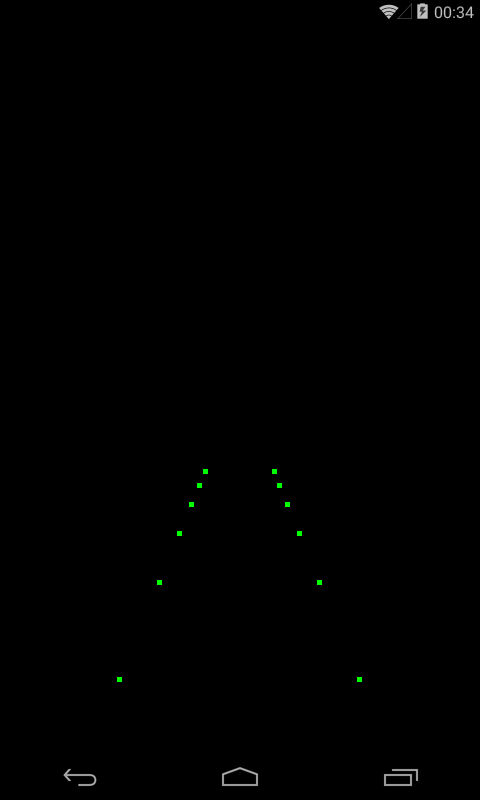Now that we have used the matrix, the z-coordinates we have specified for the vertices have started to work. And we got the points in perspective.

Let’s look at a more interesting example. Instead of points, we will draw triangles.

rewrite prepareData:

```private void prepareData() {
float z1 = -1.0f, z2 = -1.0f;

float[] vertices = {
// первый треугольник
-0.7f, -0.5f, z1,
0.3f, -0.5f, z1,
-0.2f, 0.3f, z1,

// второй треугольник
-0.3f, -0.4f, z2,
0.7f, -0.4f, z2,
0.2f, 0.4f, z2,
};

vertexData = ByteBuffer
.allocateDirect(vertices.length * 4)
.order(ByteOrder.nativeOrder())
.asFloatBuffer();
vertexData.put(vertices);
}
```

We will draw two identical triangles in size. The z-coordinates of the vertices are plotted in the variables z1 and z2 for convenience. By changing the values ​​of these variables, we will change the distance to the triangles in the 3D scene. z1 is the distance to the first triangle and z2 is to the second.

rewrite the method onDrawFrame:

```@Override
public void onDrawFrame(GL10 arg0) {
glClear(GL_COLOR_BUFFER_BIT | GL_DEPTH_BUFFER_BIT);

// зеленый треугольник
glUniform4f(uColorLocation, 0.0f, 1.0f, 0.0f, 1.0f);
glDrawArrays(GL_TRIANGLES, 0, 3);

// синий треугольник
glUniform4f(uColorLocation, 0.0f, 0.0f, 1.0f, 1.0f);
glDrawArrays(GL_TRIANGLES, 3, 3);
}
```

In the method glClear we added the variable GL_DEPTH_BUFFER_BIT. This is required to clear the depth buffer.

Next, for each triangle, we specify a color and ask the system to draw it.

Also, a string must be added at the beginning of the onSurfaceCreated method.

```glEnable(GL_DEPTH_TEST);
```

This line includes the use of depth buffer. This will allow the system to determine which point is closer to us and display it. We have already discussed this in detail a little earlier.

runWe see two identical triangles.

Now changing the parameters z1 and z2 we can change the distance to the triangles.

Draw the second triangle away

To do this, change the z-coordinate value in the method prepareData

```float z1 = -1.0f, z2 = -3.0f;
```

resultNow let’s return the second to the place, and the first will be removed

```float z1 = -2.0f, z2 = -1.0f;
```give away both

```float z1 = -3.0f, z2 = -3.0f;
```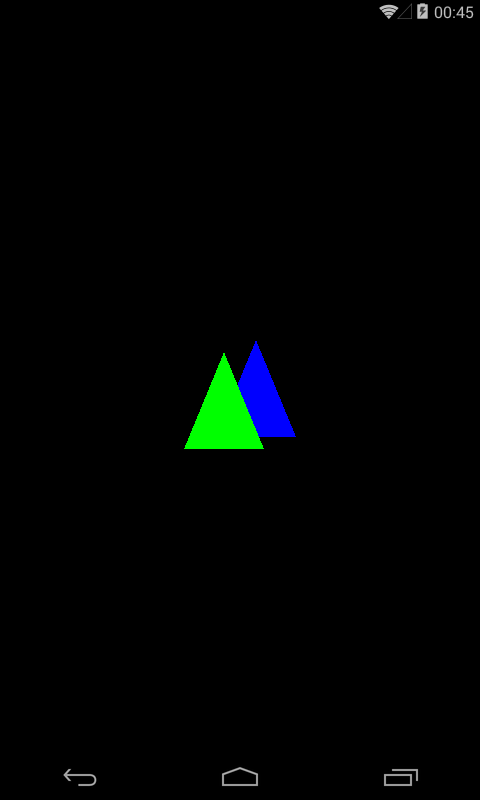The z-coordinate works as it should.

When we determined the distance to the near and far boundaries, we did so from the point (0,0,0). That’s where the default camera is. In addition, the camera is directed along the Z axis, downward (ie, as the distance from the camera the z value will decrease). We’ll learn how to change the position and direction of the camera in one of the following lessons, while we take it for granted.

Based on this information, and mentioning that near we have set = 1 and far = 8, we can assume that the camera will see all objects having z-coordinates from -1 to -8.

Let’s try to set the following values

```float z1 = -0.5f, z2 = -9.0f;
```

run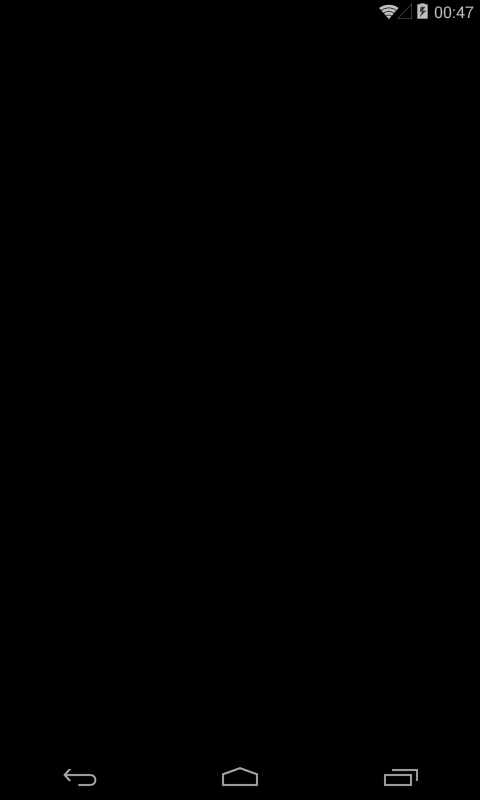Both triangles are now beyond the frustum and cannot be seen by the camera.

There is one small flaw in our matrix that we need to correct. Let’s see what the bug is about.

Set the parameters z1 and z2

```float z1 = -1.0f, z2 = -1.0f;
```

let’s launch the applicationlet’s rotate the screenIt can be seen that the picture is not the same. Let’s figure out why. 3D objects with frustum are first projected into 2D images on the near-border, and then this image from near is stretched to the real screen of the device. Near-border we have square. But the screen of the device is not square, but rectangular. And in portrait orientation, the height is greater than width, and in landscape – width is greater than height. That is, we have a square image stretched to a rectangular screen and we see a distorted picture. Corrects this easily. We just need to make the near-border aspect ratio the same as the screen aspect ratio.

That is, if we have a screen in portrait mode, for example, 480 * 800, then we divide both of these values ​​into smaller ones, that is, by 480 and get 1 * 1.66. We got the aspect ratio of the screen. And these values ​​will be used to determine the dimensions of the near-boundary. That is, in the bindMatrix method, we set left = -1, right = 1, top = 1.66, bottom = -1.66. As a result, the aspect ratio of the near-boundary will be exactly the same as the aspect ratio of the screen. And the final picture will be evenly stretched to the screen without any distortion.

Accordingly, when you turn the screen in landscape orientation, we get a resolution of 800 * 480, and the aspect ratio will be 1.66 * 1. And in bindMatrix we set left = -1.66, right = 1.66, top = 1, bottom = -1.

rewrite bindMatrix

```private void bindMatrix(int width, int height) {
float ratio = 1.0f;
float left = -1.0f;
float right = 1.0f;
float bottom = -1.0f;
float top = 1.0f;
float near = 1.0f;
float far = 8.0f;
if (width > height) {
ratio = (float) width / height;
left *= ratio;
right *= ratio;
} else {
ratio = (float) height / width;
bottom *= ratio;
top *= ratio;
}

Matrix.frustumM(mProjectionMatrix, 0, left, right, bottom, top, near, far);
glUniformMatrix4fv(uMatrixLocation, 1, false, mProjectionMatrix, 0);
}
```

We use the input width and height parameters to determine the aspect ratio and to determine the screen orientation. And depending on the orientation, we set the height and width of the near-border in proportion to the size of the screen.

runrotate the screenNow the result is the same

If for some reason you do not need perspective and full 3D, you can use ortho instead of perspective mode. The difference from ortho from perspective is that the matrix will describe not a pyramid but a parallelogram.In this mode, the subject will always be the same size, no matter how far away from the camera. If you are creating a 2D game on OpenGL, then this mode is perfect for you.

To use this mode, you just need to form the matrix using the orthoM method instead of the frustumM in bindMatrix.

Try changing the z-coordinates of the triangles yourself and make sure that the perspective no longer works.

I noticed that in ortho mode, triangles are not displayed when placed near the near-border level. That is, z = -1 in our example. I cannot yet explain why. In perspective mode, there is no such problem.

It was a difficult subject and it was quite normal that understanding would not come immediately. Just re-read this lesson periodically, and gradually everything will fall into place.

I also highly recommend that you download a demo from this page. Search for the file name there: matrixProjection.zip. Upload the archive, remove and run the exe-shnik in the bin folderYou can change projection type: perspective or ortho, and specify the parameters for creating the matrix yourself. We just talked about all this, so that you will be able to practice perfectly and clearly here.

Discuss in the forum [18 replies]